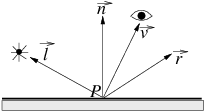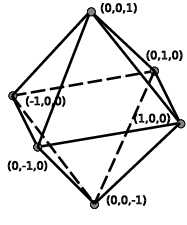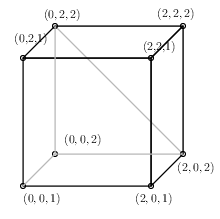UNB/ CS/ David Bremner/ teaching/ cs4735/ milestones/ Class test 2

## Practice Questions

### Lighting

Consider a point P on a flat surface, as shown on the right. Let l⃗ be the unit vector pointing to the light source, r⃗ the unit reflection vector, v⃗ the unit vector pointing to the viewer and n⃗ the unit surface normal vector at P

• How must the flat surface be tilted so that the point P has the maximum intensity diffuse reflection.

• Assume the light source and the viewer remain fixed. How must the flat surface be tilted so that the viewer receives the maximum intensity specular reflection from point P?### Articulation

Consider the articulated model “bug” illustrated below, where two arms move together (controlled by angle α), and the third is controlled by angle β. Suppose you have a function `drawSegment()` that draws a segment from (0, 0) to (1, 0), and functions `rotate(angle)`, `translate(x,y)`, `pushMatrix()` and `popMatrix()` that manipulate the model matrix. Give pseudocode to draw the model with the origin marked by , and rotation about that centre defined by a third angle γ; in the illustration γ = 0. You may assume all segments are unit length.### Textures

Give a mathematical function to map the surface of the regular octahedron onto texture coordinates 0 ≤ s ≤ 1, 0 ≤ t ≤ 1, so that the surface is covered by exactly 2 copies of the texture.### Interpolation

Suppose the near clipping plane is at z = 1. Consider the perspective projection of the triangle (0, 2, 2)(2, 0, 2)(2, 2, 2). Will it make a difference if interpolation is done before or after projection for this triangle? Why or why not?